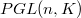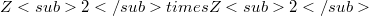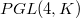﻿

### On a class of algebras

#### Abstract

We introduce the notion of projective gruppal algebra that connects to every subgroup of order n of$PGL (n, K)$ a n-dimensional algebra over K.In particular we classify the projective gruppal algebras 4-dimensional over a perfect field K studying the conjugacy classes of the groups$Z2 times Z2$ and$Z4$ in$PGL (4, K)$.

DOI Code: 10.1285/i15900932v16n2p267

Full Text: PDF